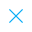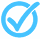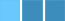Call Support +91-85588-96644

Keep me logged in
You can't leave Captcha Code empty
By submitting this form, you agree to the Terms & Privacy Policy.
ORTests given

OR# FLT - 5 Mathematics (X - 2018)

Topics Covered:Calculating Probability in Simple Independent EventsFundamental Theorem of FactorizationSurface Area of Combination of SolidsTrigonometric IdentitiesApplications of Section FormulaAreas Related to CirclesBasic Trigonometric Ratios And their ReciprocalsCalculating Mean of Grouped DataConsistency and Number of SolutionsConstruction of a Triangle Similar to a Given Triangle

This pdf is currently unavailable.
Description:
This is the practice test of Mathematics (X). It is helpful in the preparation of board exams.
Keywords: